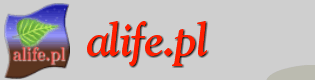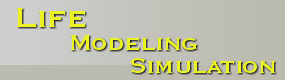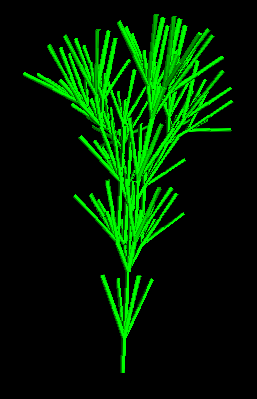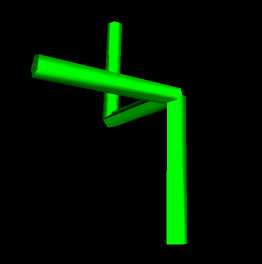# Three-dimensional L-System

Choose language of the program:

Control keys:

 Mouse left button rotate Mouse right button move

## How does it work?

3D L-Systems are an extension of the traditional 2D L-Systems. They have the ability to generate spatial forms like plants, trees or 3D mathematical shapes (a sample plant is shown below).The base rule of making L-Systems remains the same. We use the starting axiom and a set of rules which are used to modify the starting production.

However, another description of turtle state is required. Position is described by three variables (x,y,z) instead of two (x,y). The direction of turtle heading is also defined by three numbers. Each of them tells about turtle rotation around each axis.

Therefore we have to extend the set of (2D) symbols:

 F forward step f forward step without drawing + turn left by angle - turn right by angle ^ pitch up by angle & pitch down by angle / roll right by angle \ roll left by angle | turn around [ push current state of turtle onto a stack ] pop state from a stack and set as current tutrle state

Other symbols are ignored.

Thus, for production F[-F][^F/+F] and angle 90 degrees we will get:• F: forward step (vertical line in the foreground)
• -F: turn left and move forward (horizontal line in the foreground)
• ^F: pitch up and forawrd step (horizontal linie falling into background)
• /+F: roll right, turn left then forward step (vertical line in the background)

When using only F,f,+,-,[,], symbols, we get the traditional 2D L-Systems.

 Program and text: Jaroslaw Puszczynski Porting to javascript: Bartosz Tatarski Mentor: Maciej Komosinski